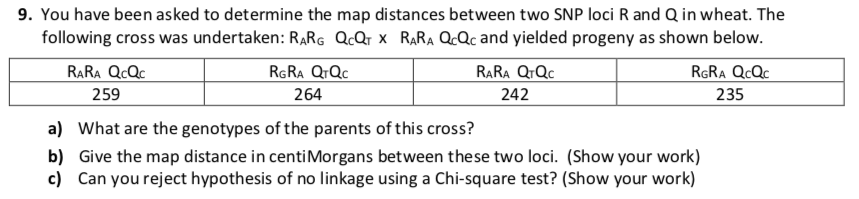# 9. You have been asked to determine the map distances between two SNP loci R and Q in wheat. The following cross was undertaken: RARG QcQrx RARA QcQc and yielded progeny as shown below. RARA QrQc RAR...9. You have been asked to determine the map distances between two SNP loci R and Q in wheat. The following cross was undertaken: RARG QcQrx RARA QcQc and yielded progeny as shown below. RARA QrQc RARA QcQc 259 235 242 264 What are the genotypes of the parents of this cross? a) Give the map distance in centiMorgans between these two loci. (Show your work) c) Can you reject hypothesis of no linkage using a Chi-square test? (Show your work) b)

Answer 1. RARG QTQC x RARA QCQC

JUSTIFICATION : The parents are the ones who are crossed to generate progeny. Therefore, the parent genotypes are the same as the genotypes mentioned in the question

Justification : the genotypes with crossing over are 242 and 235.

Sum = 242+235 = 477

Linkage distance = (477/ 1000) *100 = 47.7 cM

Answer 3. No, the hypothesis of no Linkage can not be rejected.

Justification :

If the genes are far apart on the chromosome a cross over will occur every time that pairing occurs and an equal number of parental and recombinant chromosomes will be produced. Test cross data will then generate a 1:1:1:1 ratio.

In the given case, a similar 1:1:1:1 ratio is observed that indicates that the genes are widely apart and there is no linkage between the two genes.